# DAV Class 6 Maths Chapter 5 Brain Teasers Solutions

The DAV Class 6 Maths Solutions and DAV Class 6 Maths Chapter 5 Brain Teasers Solutions of Percentage and its Applications offer comprehensive answers to textbook questions.

## DAV Class 6 Maths Ch 5 Brain Teasers Solutions

Question 1A.

(a) 2.6% expressed as a decimal is _________
(i) 0.26
(ii) 0.026
(iii) 26
(iv) 2.6
Solution:
(ii) 0.026
∴ 2.6% = $$\frac{2.6}{100}$$ = 0.026
Hence, (ii) is the correct option.

(b) What per cent is $$\frac{1}{2}$$ dozen of one score?
(i) 10%
(ii) 60%
(iii) 30%
(iv) 25%
Solution:
(iii) 30%
x% of 20 = $$\frac{1}{2}$$ × 12
$$\frac{x}{100}$$ × 20 = 6
x = $$\frac{6 \times 100}{20}$$ = 30
Hence, (iii) is the correct option.(c) If C.P. = ₹ 75, S.P. = ₹ 100, then gain or loss per cent is
(i) Gain of 33%
(ii) Loss of 33%
(iii) Loss of 25%
(iv) Gain of 25%
Solution:
When S.P. > C.P., there is a gain
Gain = S.P. – C.P.
= 100 – 75 = 25
Gain% = $$\frac{\text { Gain }}{\text { C.P. }}$$ × 100
= $$\frac{25}{75}$$ × 100
= $$\frac{100}{3}$$
= 33 $$\frac{1}{3}$$ %
Hence, (i) is the correct option.

(d) Gain or Loss percent is always calculated on
(i) SP
(ii) Loss
(iii) Gain
(iv) CP
Solution:
(iv) CP
Gain or loss per cent is always calculated in C.P.

(e) 33 $$\frac{1}{3}$$ % of 1$$\frac{1}{2}$$ minute is equal to-
(i) 90 seconds
(ii) 30 seconds
(iii) 50 seconds
(iv) 60 seconds
Solution:
(ii) 30 seconds
33 $$\frac{1}{3}$$ % of 1$$\frac{1}{2}$$
= $$\frac{100}{3} \times \frac{1}{100} \times \frac{3}{2}$$ minute
= $$\frac{1}{2}$$ × 60 sec
= 30 seconds
Hence, (ii) is the correct option.(a) What is the number which is 5% more than 600?
Solution:
600 + 5% of 600 = 600 + $$\frac{5}{100}$$ × 600
= 600 + 30 = 630

(b) Find the simple interest on ₹ 200 at 5% per annum for 6 months.
Solution:
Here. P = ₹ 200,
R = 5%,
T = 6 months
= $$\frac{1}{2}$$ year
∴ Simple interest S.I. = $$\frac{\mathrm{P} \times \mathrm{R} \times \mathrm{T}}{100}$$
= $$\frac{200 \times 5 \times 1}{100 \times 2}$$
= ₹ 5

(e) If 140 is reduced to 112, what per cent is the reduction?
Solution:
Reduction = 140 – 112 = 28
∴ Reduction % = $$\frac{\text { Reduction }}{\text { Original number }}$$ × 100
= $$\frac{28}{140}$$ × 100
= 20 %

(d) What is 12% of 0.5 metre?
Solution:
12% of 0.5 metre
= $$\frac{12}{100}$$ × 0.5 × 100 cm
= 12 × 0.5
= 6 cm

(e) If SP = ₹ 260, Profit = ₹ 60, find profit per cent.
Solution:
We know that C.P. = S.P. – Profit
∴ C.P. = ₹ 260 – ₹ 60 = ₹ 200
∴ Profit % = $$\frac{\text { Profit }}{\text { C.P. }}$$ × 100
= $$\frac{60}{100}$$ × 100
= 30%Question 2.
Find the value of:
(a) 33 $$\frac{1}{2}$$ % of 9012
Solution:
33 $$\frac{1}{3}$$ % of 9012
== 3004.

(b) 25% of 10% of 1 kg
Solution:
25% of 10% of 1 kg
=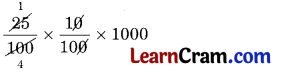= 25 gm
[∵ 1 kg = 1000 gm]

Question 3.
Find 12 $$\frac{1}{2}$$ % less than 16 hours.
Solution:
12 $$\frac{1}{2}$$ % of 16 hours
=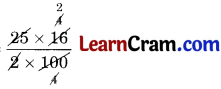= 2 hours
∴ less = 16 hours – 2 hours = 14 hours

Question 4.
John bought 100 eggs for ₹ 40. Out of these, 4 eggs were found to be broken and he sold the remaining eggs at the rate of ₹ 7.50 per dozen. Find his gain or loss percent.
Solution:
∴ Cost price of 100 eggs = ₹ 40
4 eggs were found broken
∴ Remaining eggs = 100 – 4 = 96
S.P. of 96 eggs = $$\frac{7.50 \times 96}{12}$$
= ₹ 60.00
Here, S.P. > C.P.
∴ Gain = ₹ 60 – ₹ 40 = ₹ 20
Gain percent = $$\frac{\text { gain }}{\text { C.P. }}$$ × 100
=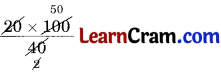= 50%
Hence, the required gain = 50%.Question 5.
Articles are bought at ₹ 45 per dozen and sold at ₹ 85 per score. Find the gain or loss per cent.
Solution:
C.P. = $$₹ \frac{45}{12}=₹ \frac{15}{4}$$
S.P. = $$₹ \frac{85}{20}=₹ \frac{17}{4}$$
Gain = S.P. – C.P.
= $$\frac{17}{4}-\frac{15}{4}$$
= $$\frac{17-15}{4}$$
= $$\frac{2}{4}=₹ \frac{1}{2}$$

Gain % = $$\frac{\text { Gain }}{\text { C.P. }}$$ × 100
= $$\frac{\frac{1}{2}}{\frac{15}{4}}$$ × 100
= $$\frac{4 \times 100}{2 \times 15}$$
= $$\frac{40}{3}$$
= 13.33%

Question 6.
Salim deposited ₹ 12000 in a finance company which pays 15% interest per year. Find the amount he is expected
to get after 4$$\frac{1}{2}$$ years.
Solution:
Here, P = ₹ 12000,
R = 15% p.a.,
T = 4$$\frac{1}{2}$$
= $$\frac{9}{2}$$ years
Simple interest S.I. = $$\frac{\mathrm{P} \times \mathrm{R} \times \mathrm{T}}{100}$$
=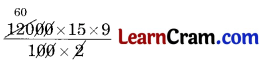= ₹ 8100

Amount = P + S.I.
= ₹ 12000 + ₹ 8100
= ₹ 20,100
Hence, the required amount = ₹ 20,100.

Question 7.
Ram Lai bought oranges at ₹ 30 per dozen. He had to sell them at a loss of 5%. Find the selling price.
Solution:
C.P. of 1 dozen oranges = ₹ 30
Loss = 5% of ₹ 30
== ₹ 1.50

∴ S.P. = C.P. – Loss
= ₹ 30 – ₹ 1.50
= ₹ 28.50
Hence, the required S.P. = ₹ 28.50.Question 8.
Out of 1200 people, 800 know only English, 50 know only Punjabi and the rest know both languages. Find the percentage of:
(a) People who know only English
(b) People who know both English and Punjabi.
Solution:
Number of people = 1200
Number of people who know English only = 800
Number of people who know Punjabi only = 50
Number of people who know both languages = 800 + 50 = 850

(a) Percentage of the people who know only English
=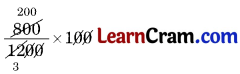= $$\frac{200}{3}$$
= 66 $$\frac{2}{3}$$ %

(b) Percentage of the people who know both the languages
=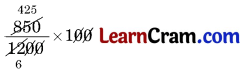= $$\frac{425}{6}$$
= 70 $$\frac{5}{6}$$ %

Question 9.
Prabal deposited ₹ 5000 in a bank which pays him 5$$\frac{1}{2}$$ % interest. After 3 years, he withdraws the money and buys an almirah for ₹ 4700. How much money is left with him?
Solution:
Here, P = ₹ 5000,
R = 5$$\frac{1}{2}$$ %
= $$\frac{1}{2}$$ %
T = 3 years
Simple interest S.I. = $$\frac{\mathrm{P} \times \mathrm{R} \times \mathrm{T}}{100}$$
= ₹ 825
Amount = P + S.I.
= ₹ 5000 + ₹ 825
= ₹ 5825
Cost of almirah = ₹ 4700
∴ Money left with him =₹ 5825 – ₹ 4700 = ₹ 1125.

Question 10.
Find the Simple Interest on ₹ 6500 at 8% from 5 January 2015 to 19 March 2015.
Solution:
P = ₹ 6500,
R = 8%
T = 27 days of Jan. + 28 days of Feb. + 18 days of March
= 73 days
= $$\frac{73}{365}$$
= $$\frac{1}{5}$$ year
S.I. = $$\frac{\mathrm{P} \times \mathrm{R} \times \mathrm{T}}{100}$$
= $$\frac{6500 \times 8 \times 1}{100 \times 5}$$
= ₹ 104### DAV Class 6 Maths Chapter 5 HOTS

Question 1.
The selling price of an article is of its cost price. Find the profit per cent.
Solution:
S.P. = $$\frac{4}{3}$$ × C.P. (given)
Profit = S.P. – C.P.
= $$\frac{4}{3}$$ C.P. – C.P.
= $$\frac{1}{3}$$ C.P.
∴ Profit % = $$\frac{\text { Profit }}{\text { C.P. }}$$ × 100
= $$\frac{\frac{1}{3} \text { C.P. }}{\text { C.P. }}$$ × 100
= $$\frac{100}{3}$$
= 33 $$\frac{1}{3}$$ %

Question 2.
In a school there are 50 students in class VIA. 88% of the students passed a Mathematics test. The same number of boys and girls failed in the exam. If 42% of the total student are girls, how many boys passed the test?
Solution:
No. of students passed in maths 88% of 50 = $$\frac{88}{100}$$ × 50 = 44
Total no. of students = 50
∴ Total no. of students failed in exam = 50 – 44 = 6
∴ No. of boys failed in exam = $$\frac{1}{2}$$ × 6 = 3
No. of girls = $$\frac{42}{100}$$ × 50 = 21
∴ No. of boys = 50 – 21 = 29
∴ No. of boys passed in exam = 29 – 3 = 26.Question 3.
The cost price of 10 articles is equal to the selling price of 8, find the Profit or loss per cent.
Solution:
C.P. of 10 articles = S.P. of 8 articles = ₹ x
∴ C.P. of 1 article = ₹ $$\frac{x}{10}$$
and S.P. of 1 article = ₹ $$\frac{x}{8}$$
∵ S.P. > C.P., there is a gain
Gain = S.P. – C.P.
= $$\frac{x}{8}-\frac{x}{10}$$
= $$\frac{5 x-4 x}{40}$$
= $$\frac{1}{40}$$ x
Profit % = $$\frac{\text { Profit }}{\text { C.P. }}$$ × 100
= $$\frac{\frac{1}{40} x}{\frac{x}{10}}$$ × 100
= $$\frac{1}{4}$$ × 100
= 25%

Question 4.
Find the number by adding 33$$\frac{1}{3}$$ % of $$\frac{1}{3}$$ of the predecessor of the smallest 5-digit number to the successor of the smallest number formed by the digits of first four composite numbers.
Solution:
Smallest 5-digit number = 10000
Predecessor of smallest 5-digit number = (10000 – 1) = 9999
Composite number are those, which has more than two different factors 4, 6, 8, 9 are the first four composite numbers
∴ Smallest numbers formed by the digits of first four composite numbers = 4689
Successor of 4689 = 4689 + 1 = 4690
As per the condition given in the question, we have
33$$\frac{1}{3}$$ % of $$\frac{1}{3}$$ of (9999) + 4690 = $$\frac{1}{3}$$ × $$\frac{1}{3}$$ × 9999 + 4690
= 1111 + 4690
= 5801### DAV Class 6 Maths Chapter 5 Enrichment Questions

Question 1.
Complete the given magic square.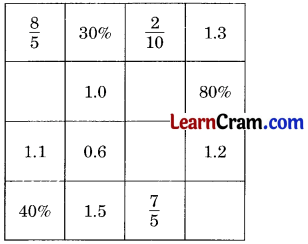Solution:Question 1.
Out of 4 dozen bananas, Ramu gave 30 of them to his younger brother. What percent of bananas he has given to him?
Solution:
Number of bananas, Ramu has = 4 × 12 = 48
Number of bananas given to his brother = 30
∴ Percentage =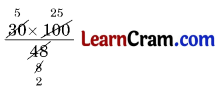= $$\frac{125}{2}$$
= 62 $$\frac{1}{2}$$ %
Hence, the required percentage = 62 $$\frac{1}{2}$$%.

Question 2.
Find the amount 8% more than 500.
Solution:
8% of 500 =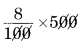= 40
∴ Amount more than 500 = 500 + 40 = 540.

Question 3.
What percent is 63 of 105?
Solution:
Percent of 63 of 105 == 60 %Question 4.
Rani got 66 marks out of 150 in Hindi. What percent marks she has got in Hindi? To get 80% in the same subject, how many marks should she get?
Solution:
Percentage of marks obtained by Rani in Hindi =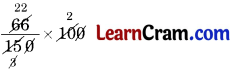= 44 %
Now 80 % of 150 =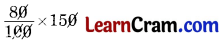= 120 marks
Hence, required marks obtained = 120.

Question 5.
Mrs. Gupta purchased a house for 4,50,000 and due to sorne reason she had to sell it for 6,00,000. Find her profit percent.
Solution:
C.P. of house = ₹ 4,50,000
S.P. of house = ₹ 6,00,000
Profit. = S.P. – C.P.
= ₹ 6,00,000 – ₹ 4,50,000 = ₹ 1,50,000
Profit percent = $$\frac{\text { Profit }}{\text { C.P. }}$$ × 100
= $$\frac{1,50,000}{4,50,000}$$
= $$\frac{100}{3}$$ %
= 33 $$\frac{1}{3}$$ %
Hence, the required profit 33 $$\frac{1}{2}$$ %.

Question 6.
Mr. Deewan deposit ₹ 50,000 in a Nationalised Bank at 5% p.a. simple interest for 2 years. Find the amount that he will get after 2 years.
Solution:
Here, P = ₹ 50,000,
R = 5% p.a.,
T = 2 $$\frac{1}{2}$$ = $$\frac{5}{2}$$ years
∴ S.I. = $$\frac{\mathrm{P} \times \mathrm{R} \times \mathrm{T}}{100}$$
=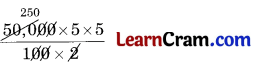= ₹ 6250
Amount = P + S.I.
= ₹ 50,000 + ₹ 6250
= ₹ 56,250
Hence, the required amount = ₹ 56,250.Question 7.
Rahul bought 50 eggs for 150. Five eggs were rotten and he sold the remaining eggs for 165. Find his loss or profit percent.
Solution:
Cost of 50 eggs = ₹ 150
Rotten eggs = 50 – 5 = 45
S.R of 45 eggs = ₹ 165
Profit = S.P. – CF.
= ₹ 165 – ₹ 150
= ₹ 15
Profit percent = $$\frac{\text { Profit }}{\text { C.P. }}$$ × 100
=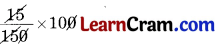Hence, the required profit percent = 10%.

Question 8.
Find the interest on ₹ 2500 at 2.5% p.a. for 146 days.
Solution:
Here, P = ₹ 2500,
R = 2.5 %
T = $$\frac{146}{365}=\frac{2}{5}$$ years
∴ Simple interest S.I. = $$\frac{\mathrm{P} \times \mathrm{R} \times \mathrm{T}}{100}$$
=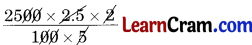= ₹ 25
Hence, the required interest = ₹ 25.

Question 9.
What percent of the multiple of 3 are in the numbers from 1 to 50?
Solution:
Multiples of 3 from 1 to 50 are 3, 6, 9, 12, 15, 18, 21, 24, 27, 30, 33, 36, 39, 42, 45, 48 i.e., 16
Required percent = $$\frac{16}{50}$$ × 100 = 32 %.Question 10.
Find the amount if ₹ 30,000 are deposited for 3 years at 10.5 percent p.a.
Solution:
Here, P = ₹ 30,000,
R = 10.5% p.a.
T = 3$$\frac{1}{2}$$
= $$\frac{7}{2}$$ years
∴ Simple interest S.I. = $$\frac{\mathrm{P} \times \mathrm{R} \times \mathrm{T}}{100}$$
=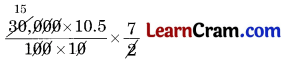= ₹ 11025
Amount = P + S.I.
= ₹ 30,000 + ₹ 11025 = ₹ 41025
Hence, the required amount = ₹ 41025.

Question 11.
Find the value of:
(a) 20% of ₹ 80
(b) 75% of ₹ 350
(c) 3 % of 180 km
(d) 100% of ₹ 1
(e) 25% of 1 score pencils
(f) 12% of kg
Solution:
(a) 20% of ₹ 80 = $$\frac{20}{100}$$ × 80 = ₹ 16
(b) 75% of ₹ 350 = $$\frac{75}{100}$$ × 350
= $$\frac{525}{2}$$
= ₹ 262.50

(c) 3$$\frac{1}{3}$$ % of 180 km
== 6 km

(d) 100 % of ₹ 1
= $$\frac{100}{100}$$ × 1
= ₹ 1

(e) 25% of 1 score pencils
== 15 pencil

(f) 12% of $$\frac{1}{2}$$ kg
== $$\frac{1}{16}$$ kgQuestion 12.
Rohan’s monthly earning is ₹ 8000. He spent 75% of his income. How much money did he spend?
Solution:
Monthly income = ₹ 8000
Spent money = $$\frac{75}{100}$$ × 8000 = ₹ 6000
Hence, the money spent = ₹ 6000.

Question 13.
Ritu scored 68% in her Mathematics test. 1f maximum marks were 50, how many marks did she score?
Solution:
Maximum marks = 50
Marks scored = 68% of 50
= $$\frac{68}{100$$ × 50 = 34
Hence the required marks obtained 34.

Question 14.
I purchased 5 1 milk and used 80% of it for making sweets. How much milk is Ieft?
Solution:
Quantity of milk purchased = 5 l
Quantity of milk used = 80% of 5 l
= $$\frac{80}{100}$$ × 5 l
= 4 l
Quantity of milk left = 5 1 – 4 l = 1 l.Question 15.
Rakesh covered a distance of 350 km. He travelled 70% by train and 28% by bus and the rest by autorickshaw. Find the distance travelled by autorickshaw.
Solution:
Distance covered by Rakesh = 350 km
Distance travelled by train = 70% of 350
= $$\frac{70}{100}$$ × 245 km
Distance travelled by bus = 28% of 350
= $$\frac{28}{100}$$ × 350 = 98 km.
Distance travelled by autorickshaw = 350 km – (245 + 98) km
= 350 km – 343 km = 7 km.
Hence the required distance = 7 km.

Question 16.
Find the amount Meena gets on depositing ₹ 1600 at 15% rate of interest for 73 days.
Solution:
Here, P = ₹ 1600,
R = 15% P.a.,
T = $$\frac{73}{365}$$ years
= $$\frac{1}{5}$$ years
∴ Simple interest S.I. = $$\frac{\mathrm{P} \times \mathrm{R} \times \mathrm{T}}{100}$$
=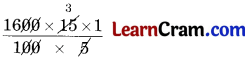= ₹ 48
Amount = P + S.I.
= ₹ 1600 + ₹ 48 = ₹ 1648
Hence the required amount deposited = ₹ 1648.Question 17.
What percent is:
(a) 5 seconds of 1 minute?
(b) 17 of 68?
Solution:
(a) 1 minute = 60 seconds
∴ 5 seconds of 60 seconds
=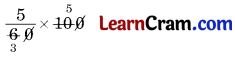= $$\frac{25}{3}$$ %
= 8 $$\frac{1}{3}$$ %

(b) 17 of 68
=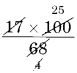= 25 %